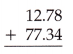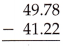# McGraw Hill Math Grade 6 Lesson 11.4 Answer Key Estimating Decimal Sums and Differences

Practice questions available in McGraw Hill Math Grade 6 Answer Key PDF Lesson 11.4 Estimating Decimal Sums and Differences will engage students and is a great way of informal assessment.

## McGraw-Hill Math Grade 6 Answer Key Lesson 11.4 Estimating Decimal Sums and Differences

Exercises Estimate

Question 1.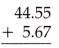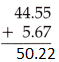The actual sum is 50.22
The estimated sum is 50.20

Question 2.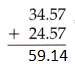The actual sum is 59.14
The estimated sum is 59.10

Question 3.The actual difference is 66.1
The estimated difference is 66

Question 4.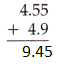The actual sum is 9.45
The estimated sum is 9.50

Question 5.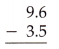The actual difference is 6.1
The estimated difference is 6

Question 6.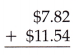The actual sum is 19.36
The estimated sum is 19.4

Question 7.The actual difference is 1.18
The estimated difference is 1.2

Question 8.Answer:$10.19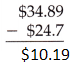The actual difference is$10.19
The estimated difference is $10.2 Question 9.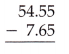Answer: 46.9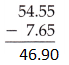The actual difference is 46.9 The estimated difference is 47 Question 10.Answer:$5.8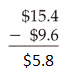The actual difference is $5.8 The estimated difference is$6

Question 11.